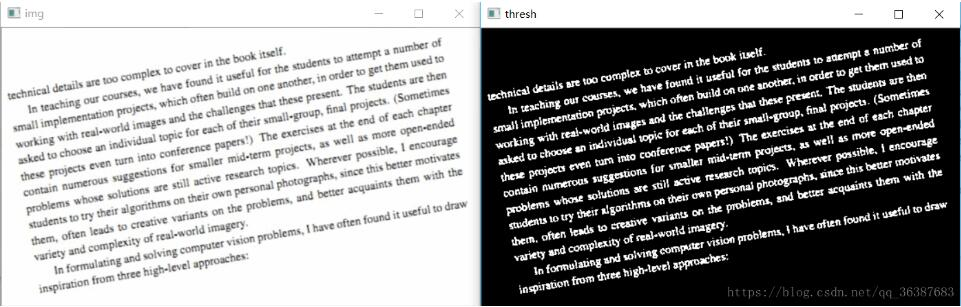# Python+OpenCV实现旋转文本校正方式

更新时间：2020年01月09日 09:41:00   转载 作者：云net

1、检测出图中的文本范围

2、计算出文本被旋转的角度

3、将图像旋转特定的角度

```#读取图像，做二值化处理
gray = cv.cvtColor(img, cv.COLOR_BGR2GRAY)
cv.imshow('gray', gray)
#像素取反，变成白字黑底
# gray = cv.bitwise_not(gray)
ret, thresh = cv.threshold(gray, 0, 255, cv.THRESH_BINARY_INV | cv.THRESH_OTSU)
cv.imshow('thresh', thresh)``````#计算包含了旋转文本的最小边框
coords = np.column_stack(np.where(thresh > 0))
print(coords)
#该函数给出包含着整个文字区域矩形边框，这个边框的旋转角度和图中文本的旋转角度一致
angle = cv.minAreaRect(coords)[-1]
print(angle)```

```#调整角度
if angle < -45:
angle = -(90+ angle)
else:
angle = -angle```

```#仿射变换
h, w = img.shape[:2]
center = (w//2, h//2)
print(angle)
M = cv.getRotationMatrix2D(center, angle, 1.0)
rotated = cv.warpAffine(img, M, (w, h), flags=cv.INTER_CUBIC, borderMode=cv.BORDER_REPLICATE)
cv.putText(rotated, 'Angle: {:.2f} degrees'.format(angle), (10, 30), cv.FONT_HERSHEY_SIMPLEX, 0.7, (0, 0, 255), 2)

print('[INFO] angel :{:.3f}'.format(angle))
cv.imshow('Input', img)
cv.imshow('Rotated', rotated)
```